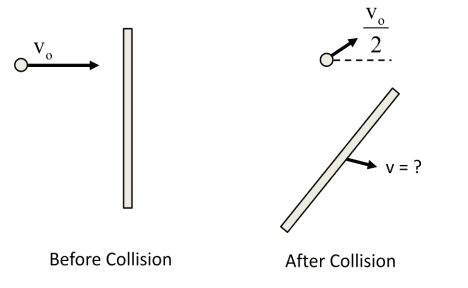# Conservation of Angular momentum and linear momentum

• WhiteG7

#### WhiteG7

I've been a fan of this forum for a while, but never signed up for it, today I'm stuck on this problem and can't find anywhere other than Chegg but I don't have a premium account.

1. Homework Statement

A meter stick is at rest on frictionless surface. A hockey puck is going towards the 30cm mark on the stick and is traveling perpendicular to the stick.
After the collision the puck is deflected 30 degrees from original path and is traveling half its original speed.

Mass of stick - 0.05kg
Mass of puck - 0.17kg
Initial speed of puck - 9 m/s

a) Choosing an origin at the starting position of the meter stick’s center of mass, what is the angular momentum of the hockey puck before the collision.
b) What is the angular momentum of the hockey puck after the collision (use the same origin)?
c) What is the velocity (direction and speed) of the stick’s center of mass after the collision?
d) What is the angular velocity of the stick (assume it will rotate about its center of mass)?## Homework Equations

L = I * w w= v/r L = r x p p = m * v
L = m * r * sin(theta) = m ( r x p )

## The Attempt at a Solution

I'm stuck on a so I didn't attempt the rest... but here is what I did for a!

L = (0.17kg) (9m/s) r sin(theta)
Theta is the angle b/t "v" and "r"

Then I multiplied the mass and velocity to get 1.53 kgm/s
Then I said in order to find the angular momentum we need to know how far the puck is from the stick's center of mass and the angle b/t v vector and r vector.

Now as I write this I think that I should have summed all the forces in x and y component but then I still don't get what to do after that...

Then I said in order to find the angular momentum we need to know how far the puck is from the stick's center of mass and the angle b/t v vector and r vector.
The problem statement doesn't say this, but it would be usual in a problem like this to calculate the ang mom of the puck immediately before the collision. That calculation is easy because the puck is at the 30cm point of the stick, and the angle between its velocity vector and the vector between stick centre of mass and puck is 90 degrees.

You can make use of the conservation of angular momentum before the collision to find a point where r and θ are easy to calculate.

D'oh! I forgot about what Dr Claude pointed out. So the problem doesn't need to tell you to calculate ang mom at the above point. You are free to choose where to calculate it because of it being conserved.

So I set the initial ang momentum equal to final ang momentum?

So I set the initial ang momentum equal to final ang momentum?
Yes.

I see one of my students is trying to get the answers for his homework 9 from online.

Regards,

Dr Fal